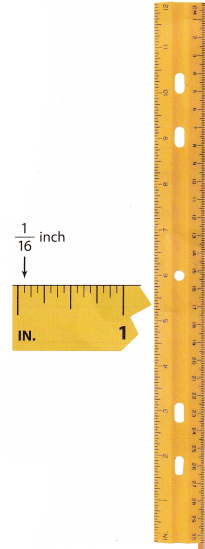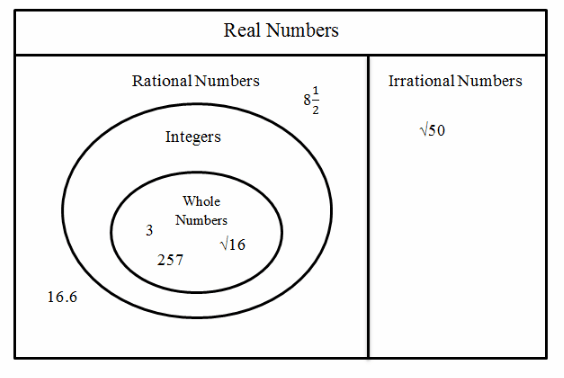# Texas Go Math Grade 8 Lesson 1.2 Answer Key Sets of Real Numbers

Refer to our Texas Go Math Grade 8 Answer Key Pdf to score good marks in the exams. Test yourself by practicing the problems from Texas Go Math Grade 8 Lesson 1.2 Answer Key Sets of Real Numbers.

## Texas Go Math Grade 8 Lesson 1.2 Answer Key Sets of Real Numbers

Essential Question
How can you describe relationships between sets of real numbers?

Write all names that apply to each number.

Question 1.
A baseball pitcher has pitched 12$$\frac{2}{3}$$ innings.12$$\frac{2}{3}$$ = $$\frac{38}{3}$$ is a fraction, so it is rational and real.

Rational, real

Question 2.
The length of the side of a square that has an area of 10 square yards. __________________
10 is a whole number, integer, real and a rational number
Length of the side of the given square is $$\sqrt{10}$$ and it is an irrational (5 is a whole number that is not a perfect square), real number.

10 – integer, whole, real, rational
$$\sqrt{10}$$ – irrational, real

Tell whether the given statement is true or false. Explain your choice.

Question 3.
All rational numbers are integers.
All rational numbers are integers

False. Every integer is a rational number but every rational number is not an integer Ration numbers such as $$\frac{3}{8}$$ and –$$\frac{7}{2}$$ are not integers.

Texas Go Math Grade 8 Pdf Lesson 1.2 Real Numbers Answer Key Question 4.
Some irrational numbers are integers.
False, every integer is a rational number (Integers are a subset of rational numbers), but no rational number ¡s an
irrational number and no irrational number is a rational number. So no irrational number is an integer.

False. Click for explanation

Identify the set of numbers that best describes the situation. Explain your choice.

Question 5.
the amount of water in a glass as it evaporates
The set of real, numbers best describes the situation since the number of water in a glass can be any number greater or even to 0.

Real numbers.

Question 6.
the number of seconds remaining when a song is playing, displayed as a negative number
Set of integers best describes the situation since seconds can’t be a fraction or an irrational number.

Integers.

Write all names that apply to each number. (Example 1)

Question 1.
$$\frac{7}{8}$$
$$\frac{7}{8}$$

Rational Number, Real

Question 2.
$$\sqrt{36}$$
$$\sqrt{36}$$ = 6
Natural Number, Rational, whole number, integer, real

Question 3.
$$\sqrt{24}$$
$$\sqrt{24}$$ is an irrational, real number

Irrational, real

Question 4.
0.75
Since 0.75 can be expressed as $$\frac{3}{4}$$ it is a rational, real number but it is not an integer.

Rational, real

Question 5.
0
Rational, Whole number, Integer, real

–$$\sqrt{100}$$
–$$\sqrt{100}$$ = -10
Rational, integer, real

Question 7.
$$5 . \overline{45}$$
Rational Numbers, real number

Question 8.
$$-\frac{18}{6}$$
$$-\frac{18}{6}$$ = -3
Rational, Integer, real

Tell whether the given statement is true or false. Explain your choice. (Example 2)

Question 9.
All whole numbers are rational numbers.
True

Explanation:
The statement is true. Whole numbers are a subset of integers, which are a subset of rational numbers. It follows
that any whole number is also a rational number.
Also notice that you can write every whole number as a rational one, by expressing it as a fraction with “1” for it
denominator.

No irrational numbers are whole numbers.
True

Explanation:
No irrational numbers are whole numbers

True Whole numbers are ration numbers.

Identify the set of numbers that best describes each situation. Explain your choice. (Example 3)

Question 11.
the change ¡n the value of an account when given to the nearest dollar
Integers.
The change can be a whole dollar amount and can be positive, negative or zero

Question 12.
the markings on a standard rulerRational
The ruler is marked every $$\frac{1}{16}$$th inch.

Essential Question Check-In

Question 13.
What are some ways to describe the relationships between sets of numbers?
There are two ways that we have been using until now to describe the relationships between sets of numbers:

• Using as scheme or a diagram like the one on page 15.
• Verbal description, for example, ‘All irrational numbers are real numbers.”

Write all names that apply to each number. Then place the numbers in the correct location on the Venn diagram.

Question 14.
$$\sqrt{9}$$ _____
Since $$\sqrt{9}$$ = 3, it is a whole number, integer, rational and real number

It should be placed inside the area for whole numbers.

Whole, integer, rational and real number. Place inside the area for whole numbers.

Lesson 1.2 Go Math 8th Grade Question 15.
257 _____
257
whole, integer, rational, real

Question 16.
$$\sqrt{50}$$ _____
$$\sqrt{50}$$
irrational, real

Question 17.
8$$\frac{1}{2}$$ _____
8$$\frac{1}{2}$$ = $$\frac{17}{2}$$ is a fraction so it is a rational number and a real number, but it is not an integer nor whole.

It should be placed inside the area for rational numbers but outside the area for integers.

Rational, real. Place inside area for rational numbers and outside of integers.

Question 18.
16.6 ______
The decimal number 16.6 means “166 tenths” so it can be expressed as a fraction: $$\frac{166}{10}$$. Therefore it is a rational, real number.

It should be placed in the area for rational numbers but outside of the area for integers.

Rational, real. Place in the area for rational numbers but outside of the area for integers.

Question 19.
$$\sqrt{16}$$ _____
$$\sqrt{16}$$ = 4
Rational, whole, integers, realIdentify the set of numbers that best describes each situation. Explain your choice.

the height of an airplane as it descends to an airport runway
Set of real numbers best describes the given situation because the height of the airplane is a real number larger
than or equal to 0. The plane doesn’t jump from one height to the other, for example from 100 to 99, rather it descends gradually hitting every height on its way down.

Real numbers.

Question 21.
the score with respect to par of several golfers: 2, -3, 5, 0, -1
Integers. The scores are counting numbers, their opposites and zero.

Question 22.
Critique Reasoning Ronald states that the number $$\frac{1}{11}$$ is not rational because, when converted into a decimal, it does not terminate. Nathaniel says it is rational because it is a fraction. Which boy is correct? Explain.
Nathaniel is correct, if a number can be expressed as a ratio in the form $$\frac{a}{b}$$ where a and b are integers and b is not 0, it is a rational number.
Obviously in this case a = 1 and b = 11, so both are integers and b ≠ 0

Nathaniel is correct.

Question 23.
Critique Reasoning The circumference of a circular region is shown. What type of number best describes the diameter of the circle? Explain your answer.The formula for the circumference of a circular region is C = 2rπ where r is the radius of the circle.
C = 2rπ
π = 2rπ Substitute C = π
1 = 2r Divide both sides of the equation with π

Since the diameter of the circle is twice the length of its radius: d = 2r we get d = 1. Therefore, the type of
number that best describes the diameter of the circle is a whole number

Whole number

Question 24.
Critical Thinking A number is not an integer. What type of number can it be?
Since it is not an integer it can not be a whole number because every whole number is also an integer. It can be
any other type of number so: rational or irrational real number

Rational or irrational real number.

Simple Solutions Math Grade 8 Answer Key Pdf Lesson 1 Question 25.
A grocery store has a shelf with half-gallon containers of milk. What type of number best represents the total number of gallons?
$$\frac{1}{2}$$ gallons
Rational number

Texas Go Math Grade 8 Lesson 1.2 H.O.T. Focus On Higher Order Thinking Answer Key

Question 26.
Explain the Error Katie said, “Negative numbers are integers.” What was her error?
Her error is that not alt negative numbers are integers, for example, the number –$$\frac{1}{2}$$ is obviously a negative number but it is not an integer.

Not all negative numbers are Integers.

Question 27.
Justify Reasoning Can you ever use a calculator to determine if a number is rational or irrational? Explain.
Yes, in some cases you can use a calculator to tell if the number is rational or not
For example, if we wonder if the number 0.5 is rational we can just type 0.5 in the calculator and it will give us a
result $$\frac{1}{2}$$”. Since we have a representation of the number 0.5 as a ratio of two integers we can conclude that it is in fact a rational number

This method doesn’t always work since calculators show a limited number of digits and sometimes that is not
enough to tell it there is a repeating decimal or not.

Yes, but not always.

Draw Conclusions The decimal $$0 . \overline{3}$$ represents $$\frac{1}{3}$$. What type of number best describes $$0 . \overline{9}$$, which is 3 ∙ $$0 . \overline{3}$$? Explain.
Let
x = $$0 . \overline{9}$$
10x = 10 × $$0 . \overline{9}$$. Since $$0 . \overline{9}$$ has one repeating decimal, multiply both sides with 10
10x = $$9 . \overline{9}$$. Subtract x = $$0 . \overline{9}$$ from both sides
9x = 9
x = 1
Since x = 1 the type of number that best describes $$0 . \overline{9}$$ is a whole number.

A whole number.

Question 29.
Communicate Mathematical Ideas Irrational numbers can never be precisely represented in decimal form. Why is this?
We say that a number is irrational if it not rational, so if it can’t be expressed as a ratio $$\frac{a}{b}$$ where a and b are integers and b is not 0.
have digits repeating forever, for example $$\frac{1}{3}$$ = $$0 . \overline{3}$$, so the digit 3 goes on forever, irrational numbers don’t have a ‘rule” that we can observe. This means that to know what digit is on any place behind the decimal point we have to calculate it (where in the previous example we know that if we express the number $$\frac{1}{3}$$ as a decimal we can say with certainty exactly what digit is in what place, even if in this case it is very simple because all digits are 3)
For example $$\sqrt{2}$$ ≈ 1.414213562…